•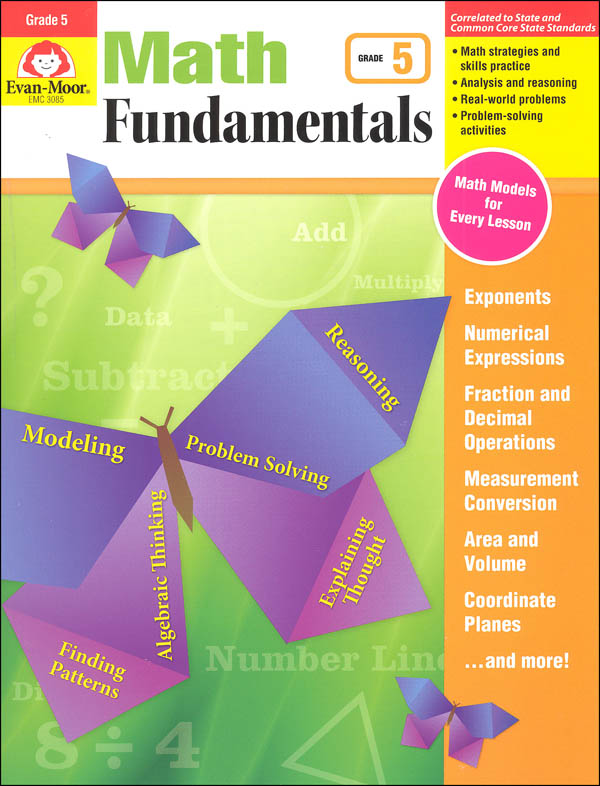•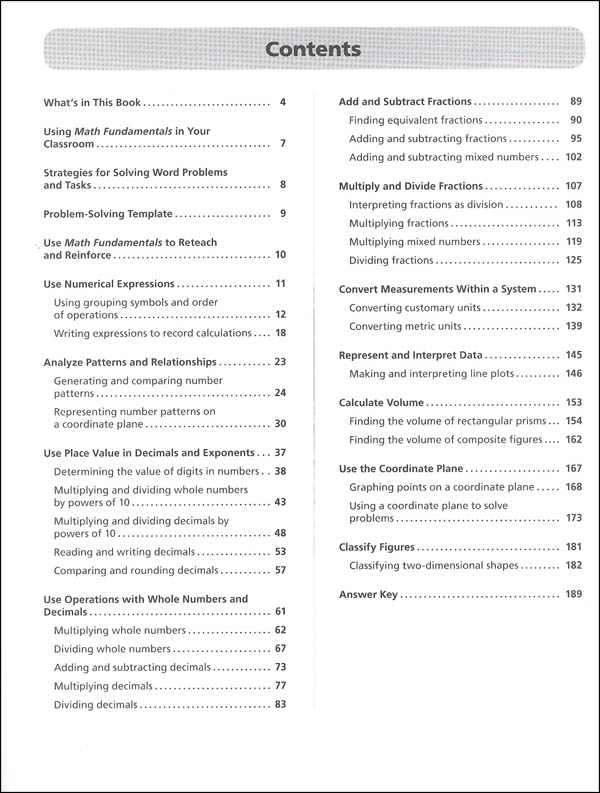•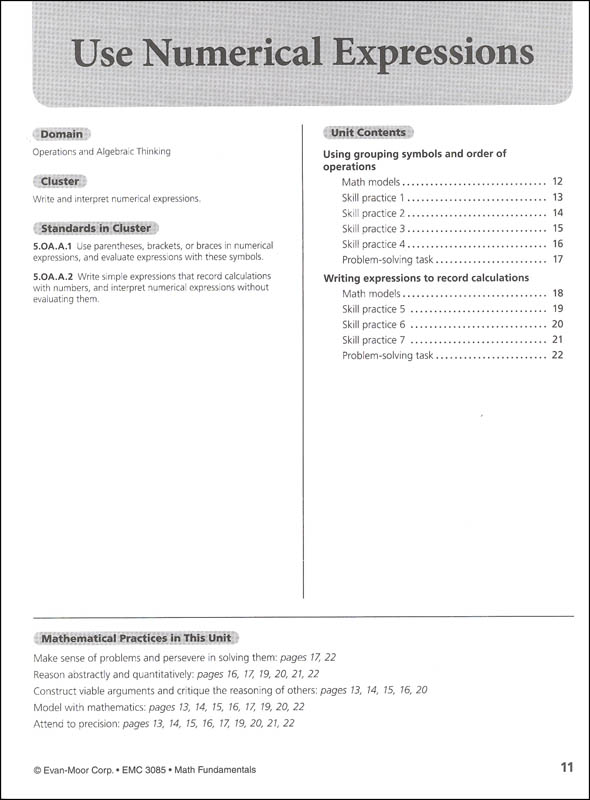•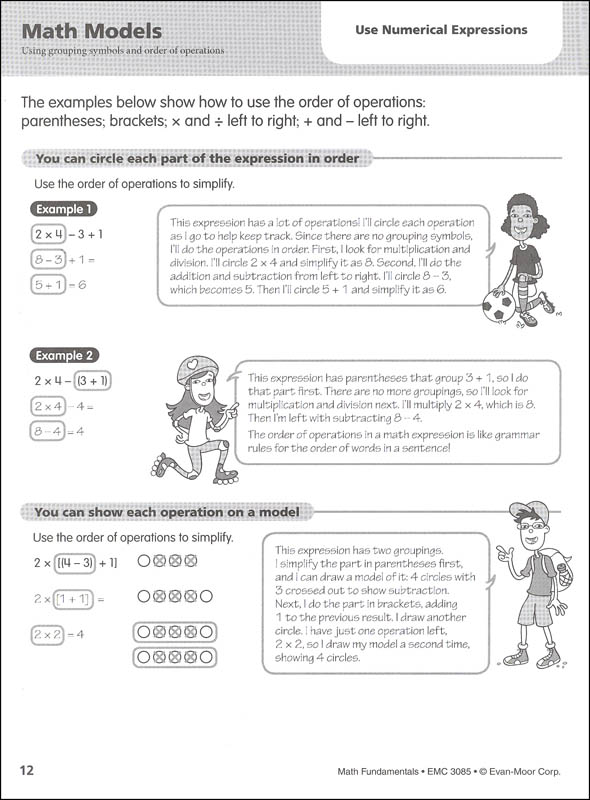•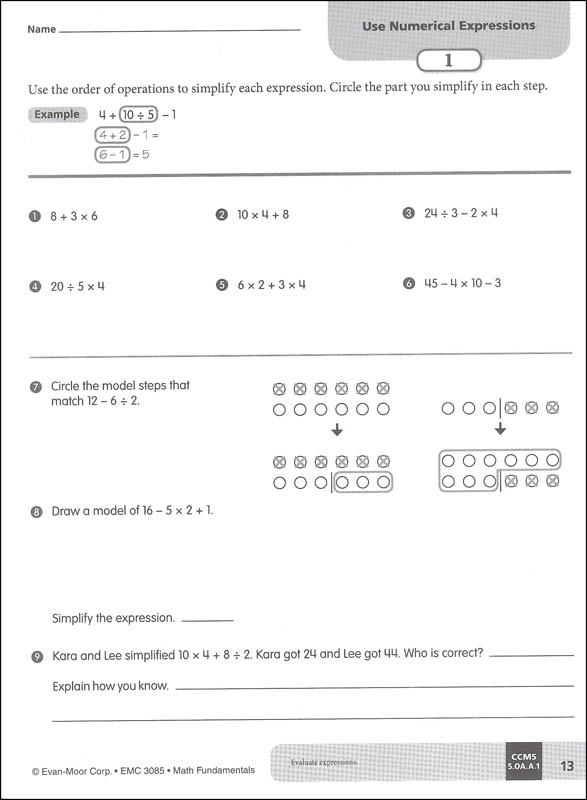•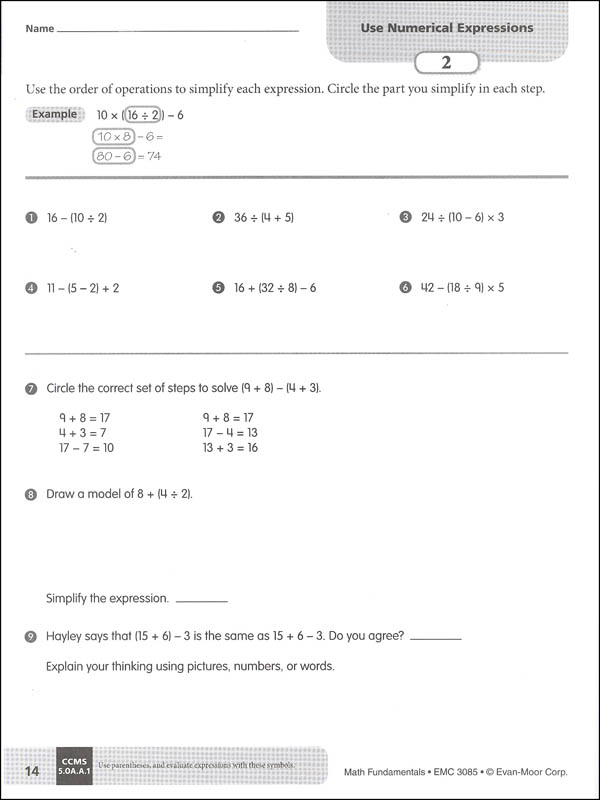•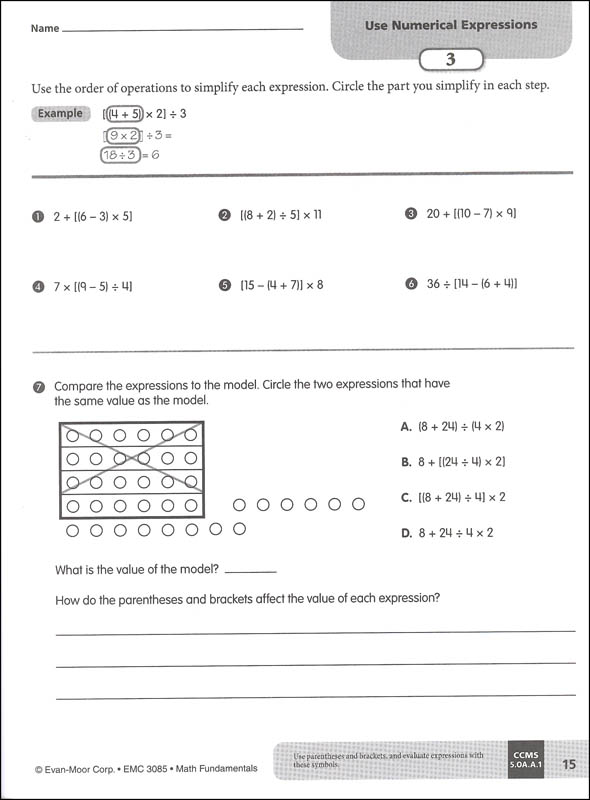•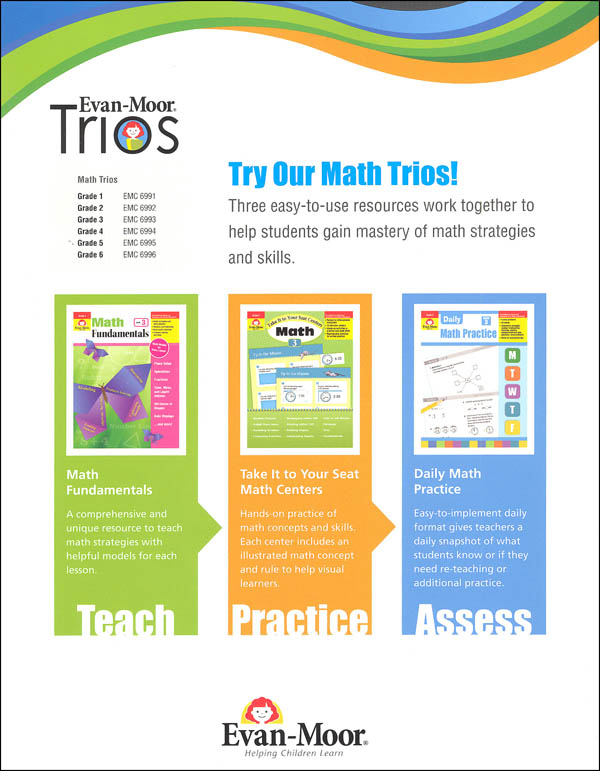# 041141

Our Price: \$13.95
Retail: \$19.99
Save: 30.22% (\$6.04)
In Stock.
Qty:
Qty:

 Item #: 041141 9781629383316 5

#### Product Description:

Exponents, numerical expressions, fraction and decimal operations, measurement conversion, area, volume, coordinate planes, classifying two-dimensional shapes.

#### Publisher Description:

Comprehensive but not complicated!Math Fundamentalshelps your fifth graders navigate the new math. Math Models and analysis questions, plenty of skill practice, and real-world problems guide students in thinking through and solving problems.

To help you target instruction, each unit clearly lists the standards information, mathematical practices, and skills covered.

Within a unit, math lessons are presented simply. Every math lesson includes:

• A Math Models reference pagethat shows students strategies for solving problems. This is helpful for parents and students transitioning to new math.
• Skill practice pagesthat progress in difficulty.
• A culminating problem-solving taskthat leads students through the process of solving a real-life problem.

• Use numerical expressions
• Analyze patterns and relationships
• Use place value in decimals and exponents
• Use operations with whole numbers and decimals
• Multiply and divide fractions
• Convert measurements within a system
• Represent and interpret data
• Calculate volume
• Use the coordinate plane
• Classify figures

Designed to support new math programs,Math Fundamentalsis the perfect easy-to-use supplement to your Common Core math or current math curriculum.

Better together! UseMath FundamentalswithDaily Math Practicefor complementary skill practice that ensures your students master grade-level math.

This resource contains teacher support pages, reproducible student pages, and an answer key.

Category Description for Math Fundamentals:

Practice problem solving skills using modeling, finding patterns, and reasoning. For use in the classroom or at home, these books can help make sense of using models to solve math problems. Aligned to state and common core standards, each level of this series is intended as a resource to helps with some of the more complicated or confusing strategies that are used in math today.

Each book is divided into topical units and the beginning of each lists the standards being met. You could work through the book in the order the pages are presented, but also might take a more topical approach to add practice to complement your curriculum. The front of the book also offers a suggested teaching path, listing the order in which to use the units and page numbers. The format is the same for each lesson within the units - a page of math models, example, practice problems, then real world problem. 'Math Models' pages offer possible strategies for solving a problem - draw a picture, draw a diagram, use counters, numberline, or write a number sentence. "Think" is an opportunity for students to explain their thinking. "Example" shows students how to mark an answer and show their work. 'Practice Problems' are an opportunity for students to practice when they just learned. 'Real-world Problem' takes students step-by-step through the problem-solving process for a written-out word problem. They will read the problem, answer thinking questions, then solve the problem using one of the strategies presented earlier. Word problems are one of the biggest challenges for students and this series offers some usable solutions. Each book is over 220 pages and is reproducible for family or classroom use. ~ Donna

Primary Subject
Mathematics
5
ISBN
9781629383316
Format
Paperback
Brand Name
Evan-Moor
Weight
1.35 (lbs.)
Dimensions
10.88" x 8.38" x 0.5"